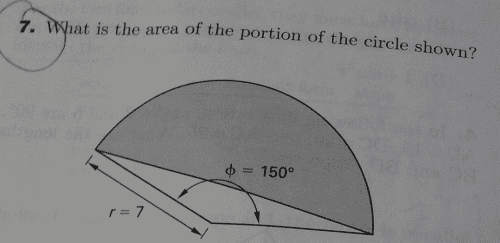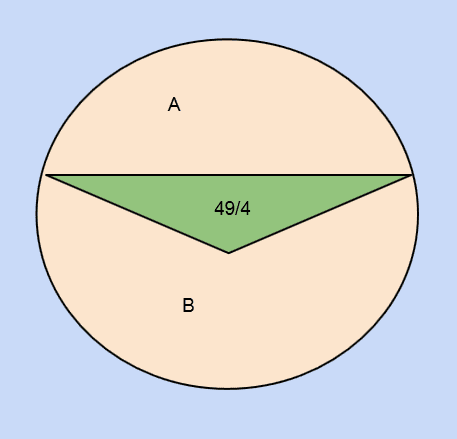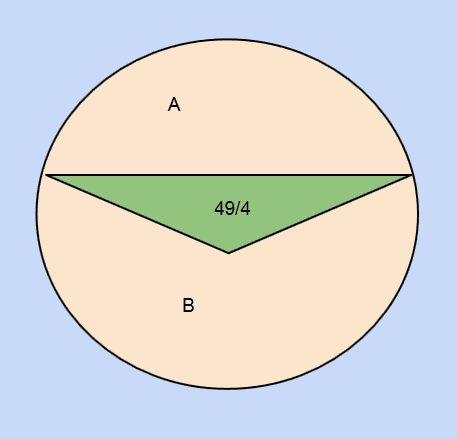# Area portion of circle

Hi all, I am having an issue trying to solve the following problem

## Homework StatementI know that the radius of the circle is 7 and the angle of the segment is 150°

## Homework Equations

Area of a circle: $$A = \pi{r}^2$$
Area of the sector of the circle: $$A = \frac{n}{360}\pi r^{2}$$
Area of a triangle: $$A = \frac{n}{360}\pi r^{2}$$

## The Attempt at a Solution

I know to get the final solution to get the area of that particular part of the circle, I need to subtract the whole area of the circle with the segment of the circle (the triangular portion).

To get the area of the triangle portion, I subtract the area of the sector of the circle with the area of the triangle of that portion multiplied by 2 (since there are essentially two triangles). Also, to get the area of the triangular portion, I can use the 30-60-90 rule. To get this:
Short leg:
$$A_s = \frac{1}{2}H = \frac{1}{2}*7 = \frac{7}{2}$$
Long leg:
$$A_l=\frac{1}{2}H\sqrt{3} = \frac{7}{2}\sqrt{3}$$
Area of whole triangle:
$$A_t=\frac{1}{2}*\frac{7}{2}*\frac{7}{2}\sqrt{3}*2=\frac{49}{4}\sqrt{3}$$

Now the area of the whole sector is:
$$A_t = \frac{150}{360}49\pi-\frac{49}{4}\sqrt{3}$$

To get the area of just that portion of the circle the question is asking, subtract the area of the whole circle with the answer from above:
$$A = 49\pi-(\frac{150}{360}49\pi-\frac{49}{4}\sqrt{3})$$

I was wondering if I am doing this problem correctly? I am not getting any of the answers in the possible solutions. Thanks!

## Answers and Replies

LCKurtz
Science Advisor
Homework Helper
Gold Member
Hi all, I am having an issue trying to solve the following problem

## Homework StatementI know that the radius of the circle is 7 and the angle of the segment is 150°

## Homework Equations

Area of a circle: $$A = \pi{r}^2$$
Area of the sector of the circle: $$A = \frac{n}{360}\pi r^{2}$$
Area of a triangle: $$A = \frac{n}{360}\pi r^{2}$$

## The Attempt at a Solution

I know to get the final solution to get the area of that particular part of the circle, I need to subtract the whole area of the circle with the segment of the circle (the triangular portion).

To get the area of the triangle portion, I subtract the area of the sector of the circle with the area of the triangle of that portion multiplied by 2 (since there are essentially two triangles). Also, to get the area of the triangular portion, I can use the 30-60-90 rule. To get this:
Short leg:
$$A_s = \frac{1}{2}H = \frac{1}{2}*7 = \frac{7}{2}$$

But that half triangle is not a 30-60-90 triangle.

But that half triangle is not a 30-60-90 triangle.

Hmmm, yes you are right. Looks like I made a mistake. Can I assume it is a right triangle if I cut it in half?

verty
Homework Helper
Hmmm, yes you are right. Looks like I made a mistake. Can I assume it is a right triangle if I cut it in half?

You are given that one leg of the triangle is a radius, that is enough to be sure the triangle is isosceles.

•1 person
LCKurtz
Science Advisor
Homework Helper
Gold Member
Hmmm, yes you are right. Looks like I made a mistake. Can I assume it is a right triangle if I cut it in half?

Yes, but why would you want to deal with angles like ##15^\circ##? What is the formula for area of a triangle given two sides and the included angle ##\theta##? Draw a picture and figure it out if you don't know the formula. It's what you need here.

•1 person
Yes, but why would you want to deal with angles like ##15^\circ##? What is the formula for area of a triangle given two sides and the included angle ##\theta##? Draw a picture and figure it out if you don't know the formula. It's what you need here.

Thanks. I researched this and it looks like I can use the SAS (Side Angle Side) formula to get the area of this triangle. That formula is:
$$A=\frac{1}{2}ab\sin C$$
Which, for my problem, is equal to:
$$A_t = \frac{1}{2}(7)(7)\sin 150\degree$$
$$A_t = \frac{1}{2}(49)(0.5)$$
$$A_t = \frac{49}{4}$$

Now to get the area of that small portion of the circle, I take the area of the whole circle and subtract it with the area of the triangle:
$$A = 49\pi - \frac{49}{4}$$
$$A = 49(\pi - \frac{1}{4})$$

Does this look right because this answer does not equal any of the possible answers for the problem. Thanks again!

In your expression A=49π−49/4, you have taken the area of the whole circle, and not a fraction of it.

•1 person
In your expression A=49π−49/4, you have taken the area of the whole circle, and not a fraction of it.

Thanks for the reply pongo38. I might be confusing myself. If I take area of the whole circle and subtract it with the area of the triangle, won't that give me the area of the smaller circle?

I think it would help if you drew the whole circle, then the 150 degree segment, then the triangle. Put on the diagram all the areas you can identify.

•1 person
I think it would help if you drew the whole circle, then the 150 degree segment, then the triangle. Put on the diagram all the areas you can identify.

Thanks pongo. I drew it out and understand why my thinking was incorrect on trying to subtract the whole circle.

So I drew it out:Now I am confused on how to continue to approach this problem. I am not sure if the the portion of (B) is exactly a half circle, which I cannot assume given the problem. I even tried to work it out and didn't get the correct problem with the same thinking. Any suggestions? Thanks

SteamKing
Staff Emeritus
Science Advisor
Homework Helper
Go back to your original post. In the Relevant equations section, you have stated the correct formula for the area of a circular sector. The formula for the area of the triangle is incorrect.

From the diagram, the area of the shaded portion = the area of the circular sector - the area of the triangle.

You have calculated the area of the circular sector as (150/360)*49 * pi
You have calculated the area of the triangle as 49/4

Can you calculate the area of the shaded portion now? Remember to simplify fractions in your final result.

•1 person
LCKurtz
Science Advisor
Homework Helper
Gold Member
Thanks pongo. I drew it out and understand why my thinking was incorrect on trying to subtract the whole circle.

So I drew it out:Now I am confused on how to continue to approach this problem. I am not sure if the the portion of (B) is exactly a half circle, which I cannot assume given the problem. I even tried to work it out and didn't get the correct problem with the same thinking. Any suggestions? Thanks

Area B has nothing to do with the problem. Find the area of the sector of the circle bounded by the two radii and subtract the area of the triangle from it.

•1 person